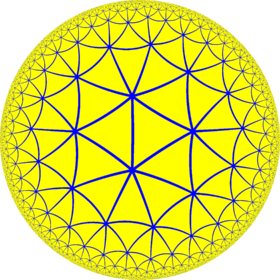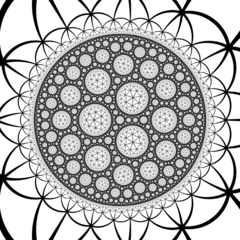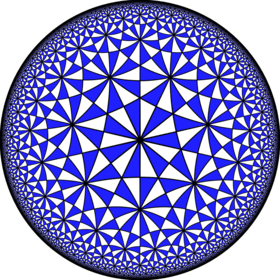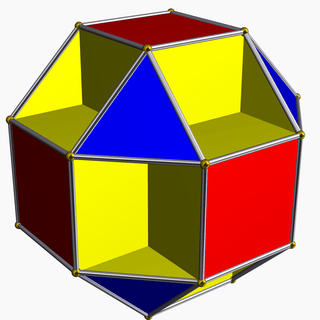# Order-7 triangular tiling

Order-7 triangular tilingPoincaré disk model of the hyperbolic plane
TypeHyperbolic regular tiling
Vertex figure37
Schläfli symbol{3,7}
Wythoff symbol7 | 3 2
Coxeter diagramSymmetry group[7,3], (*732)
DualHeptagonal tiling
PropertiesVertex-transitive, edge-transitive, face-transitive

In geometry, the order-7 triangular tiling is a regular tiling of the hyperbolic plane with a Schläfli symbol of {3,7}.The {3,3,7} honeycomb has {3,7} vertex figures.

## Hurwitz surfaces

Further information: Hurwitz surface

The symmetry group of the tiling is the (2,3,7) triangle group, and a fundamental domain for this action is the (2,3,7) Schwarz triangle. This is the smallest hyperbolic Schwarz triangle, and thus, by the proof of Hurwitz's automorphisms theorem, the tiling is the universal tiling that covers all Hurwitz surfaces (the Riemann surfaces with maximal symmetry group), giving them a triangulation whose symmetry group equals their automorphism group as Riemann surfaces.

The smallest of these is the Klein quartic, the most symmetric genus 3 surface, together with a tiling by 56 triangles, meeting at 24 vertices, with symmetry group the simple group of order 168, known as PSL(2,7). The resulting surface can in turn be polyhedrally immersed into Euclidean 3-space, yielding the small cubicuboctahedron.

The dual order-3 heptagonal tiling has the same symmetry group, and thus yields heptagonal tilings of Hurwitz surfaces.The symmetry group of the order-7 triangular tiling has fundamental domain the (2,3,7) Schwarz triangle, which yields this tiling.The small cubicuboctahedron is a polyhedral immersion of the Klein quartic, which, like all Hurwitz surfaces, is a quotient of this tiling.

It is related to two star-tilings by the same vertex arrangement: the order-7 heptagrammic tiling, {7/2,7}, and heptagrammic-order heptagonal tiling, {7,7/2}.

This tiling is topologically related as a part of sequence of regular polyhedra with Schläfli symbol {3,p}.

From a Wythoff construction there are eight hyperbolic uniform tilings that can be based from the regular heptagonal tiling.

Drawing the tiles colored as red on the original faces, yellow at the original vertices, and blue along the original edges, there are 8 forms.Wikimedia Commons has media related to Order-7 triangular tiling.